## 2.目录

1. Boosing是怎么工作的？
2. 理解GBM模型中的参数
3. 学会调参（附详例）

## 3.Boosting是如何工作的？

Boosting可以将一系列弱学习因子（weak learners）相结合来提升总体模型的预测准确度。在任意时间t，根据t-1时刻得到的结果我们给当前结果赋予一个权重。之前正确预测的结果获得较小权重，错误分类的结果得到较大权重。回归问题的处理方法也是相似的。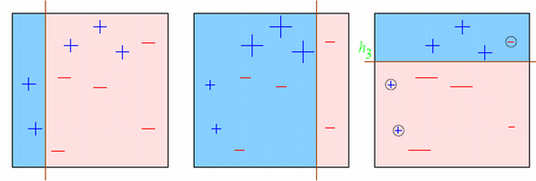1. 图一： 第一个弱学习因子的预测结果（从左至右）
• 一开始所有的点具有相同的权重（以点的尺寸表示）。
• 分类线正确地分类了两个正极和五个负极的点。
2. 图二： 第二个弱学习因子的预测结果
• 在图一中被正确预测的点有较小的权重（尺寸较小），而被预测错误的点则有较大的权重。
• 这时候模型就会更加注重具有大权重的点的预测结果，即上一轮分类错误的点，现在这些点被正确归类了，但其他点中的一些点却归类错误。

## 4.GBM参数

1. 树参数：调节模型中每个决定树的性质
2. Boosting参数：调节模型中boosting的操作
3. 其他模型参数：调节模型总体的各项运作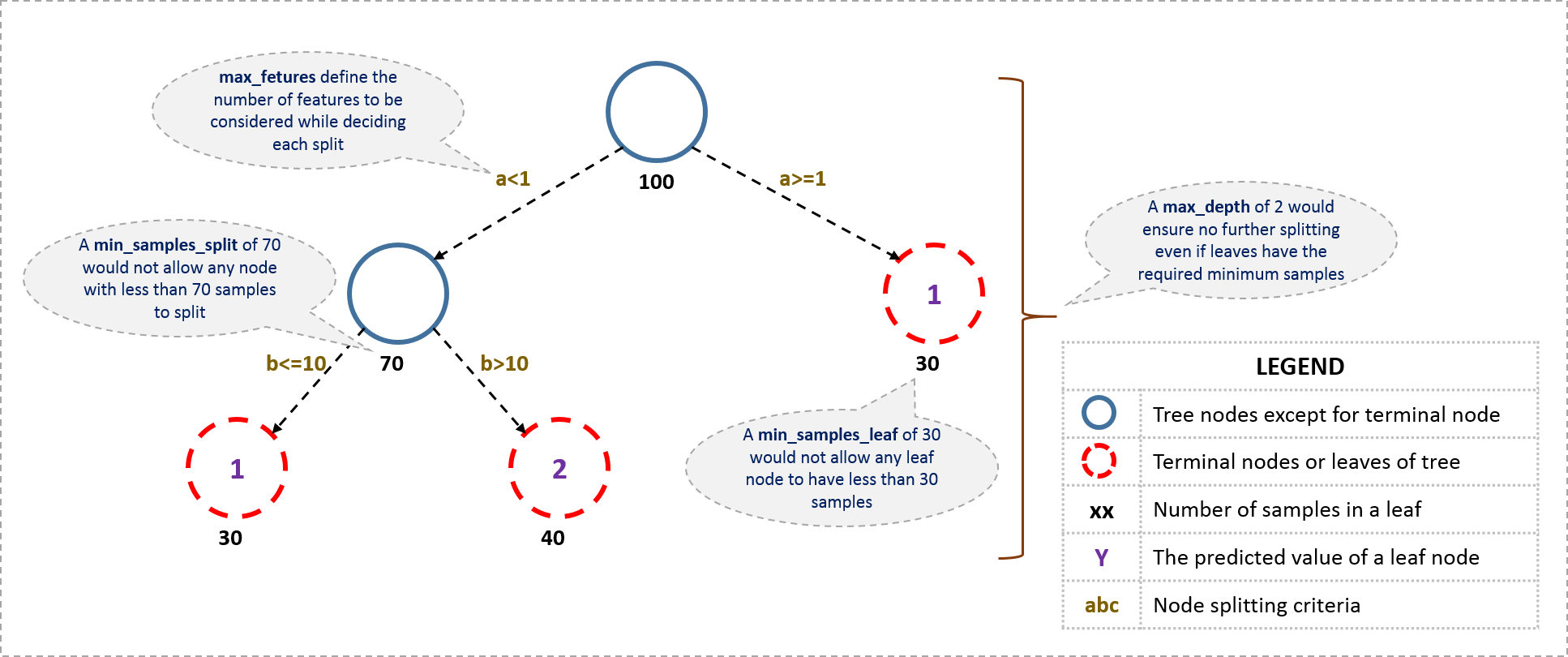1. min_ samples_split
• 定义了树中一个节点所需要用来分裂的最少样本数。
• 可以避免过度拟合(over-fitting)。如果用于分类的样本数太小，模型可能只适用于用来训练的样本的分类，而用较多的样本数则可以避免这个问题。
• 但是如果设定的值过大，就可能出现欠拟合现象(under-fitting)。因此我们可以用CV值（离散系数）考量调节效果。
2. min_ samples_leaf
• 定义了树中终点节点所需要的最少的样本数。
• 同样，它也可以用来防止过度拟合。
• 在不均等分类问题中(imbalanced class problems)，一般这个参数需要被设定为较小的值，因为大部分少数类别（minority class）含有的样本都比较小。
3. min_ weight_ fraction_leaf
• 和上面min_ samples_ leaf很像，不同的是这里需要的是一个比例而不是绝对数值：终点节点所需的样本数占总样本数的比值。
• #2和#3只需要定义一个就行了
4. max_ depth
• 定义了树的最大深度。
• 它也可以控制过度拟合，因为分类树越深就越可能过度拟合。
• 当然也应该用CV值检验。
5. max_ leaf_ nodes
• 定义了决定树里最多能有多少个终点节点。
• 这个属性有可能在上面max_ depth里就被定义了。比如深度为n的二叉树就有最多2^n个终点节点。
• 如果我们定义了max_ leaf_ nodes，GBM就会忽略前面的max_depth。
6. max_ features
• 决定了用于分类的特征数，是人为随机定义的。
• 根据经验一般选择总特征数的平方根就可以工作得很好了，但还是应该用不同的值尝试，最多可以尝试总特征数的30%-40%.
• 过多的分类特征可能也会导致过度拟合。

1. 初始分类目标的参数值
2. 对所有的分类树进行迭代：
2.1 根据前一轮分类树的结果更新分类目标的权重值（被错误分类的有更高的权重）
2.2 用训练的子样本建模
2.3 用所得模型对所有的样本进行预测
2.4 再次根据分类结果更新权重值
3. 返回最终结果

1. learning_ rate
• 这个参数决定着每一个决定树对于最终结果（步骤2.4）的影响。GBM设定了初始的权重值之后，每一次树分类都会更新这个值，而learning_ rate控制着每次更新的幅度。
• 一般来说这个值不应该设的比较大，因为较小的learning rate使得模型对不同的树更加稳健，就能更好地综合它们的结果。
2. n_ estimators
• 定义了需要使用到的决定树的数量（步骤2）
• 虽然GBM即使在有较多决定树时仍然能保持稳健，但还是可能发生过度拟合。所以也需要针对learning rate用CV值检验。
3. subsample

• 训练每个决定树所用到的子样本占总样本的比例，而对于子样本的选择是随机的。
• 用稍小于1的值能够使模型更稳健，因为这样减少了方差。
• 一把来说用~0.8就行了，更好的结果可以用调参获得。

好了，现在我们已经介绍了树参数和boosting参数，此外还有第三类参数，它们能影响到模型的总体功能：

4. loss

• 指的是每一次节点分裂所要最小化的损失函数(loss function)
• 对于分类和回归模型可以有不同的值。一般来说不用更改，用默认值就可以了，除非你对它及它对模型的影响很清楚。
5. init
• 它影响了输出参数的起始化过程
• 如果我们有一个模型，它的输出结果会用来作为GBM模型的起始估计，这个时候就可以用init
6. random_ state
• 作为每次产生随机数的随机种子
• 使用随机种子对于调参过程是很重要的，因为如果我们每次都用不同的随机种子，即使参数值没变每次出来的结果也会不同，这样不利于比较不同模型的结果。
• 任一个随即样本都有可能导致过度拟合，可以用不同的随机样本建模来减少过度拟合的可能，但这样计算上也会昂贵很多，因而我们很少这样用
7. verbose
• 决定建模完成后对输出的打印方式：
• 0：不输出任何结果（默认）
• 1：打印特定区域的树的输出结果
• >1：打印所有结果
8. warm_ start
• 这个参数的效果很有趣，有效地使用它可以省很多事
• 使用它我们就可以用一个建好的模型来训练额外的决定树，能节省大量的时间，对于高阶应用我们应该多多探索这个选项。
9. presort
• 决定是否对数据进行预排序，可以使得树分裂地更快。
• 默认情况下是自动选择的，当然你可以对其更改

## 5.参数调节实例

• City这个变量已经被我舍弃了，因为有太多种类了。
• DOB转为Age|DOB,舍弃了DOB
• 创建了EMI_Loan_Submitted_Missing这个变量，当EMI_Loan_Submitted 变量值缺失时它的值为1，否则为0。然后舍弃了EMI_Loan_Submitted
• EmployerName的值也太多了，我把它也舍弃了
• Existing_EMI的缺失值被填补为0（中位数），因为只有111个缺失值
• 创建了Interest_Rate_Missing变量，类似于#3，当Interest_Rate有值时它的值为0，反之为1，原来的Interest_Rate变量被舍弃了
• Loan_Amount_AppliedLoan_Tenure_Applied的中位数填补了缺失值
• 创建了Loan_Amount_Submitted_Missing变量，当Loan_Amount_Submitted有缺失值时为1，反之为0，原本的Loan_Amount_Submitted变量被舍弃
• 创建了Loan_Tenure_Submitted_Missing变量，当Loan_Tenure_Submitted有缺失值时为1，反之为0，原本的Loan_Tenure_Submitted变量被舍弃
• 舍弃了LoggedIn,和Salary_Account
• 创建了Processing_Fee_Missing变量，当Processing_Fee有缺失值时为1，反之为0，原本的Processing_Fee变量被舍弃
• Source-top保留了2个，其他组合成了不同的类别
• 对一些变量采取了数值化和独热编码（One-Hot-Coding）操作

#Import libraries:
import pandas as pd
import numpy as np
from sklearn.ensemble import GradientBoostingClassifier  #GBM algorithm
from sklearn import cross_validation, metrics   #Additional scklearn functions
from sklearn.grid_search import GridSearchCV   #Perforing grid search

import matplotlib.pylab as plt
%matplotlib inline
from matplotlib.pylab import rcParams
rcParams['figure.figsize'] = 12, 4

target = 'Disbursed'
IDcol = 'ID'

def modelfit(alg, dtrain, predictors, performCV=True, printFeatureImportance=True, cv_folds=5):
#Fit the algorithm on the data
alg.fit(dtrain[predictors], dtrain['Disbursed'])

#Predict training set:
dtrain_predictions = alg.predict(dtrain[predictors])
dtrain_predprob = alg.predict_proba(dtrain[predictors])[:,1]

#Perform cross-validation:
if performCV:
cv_score = cross_validation.cross_val_score(alg, dtrain[predictors], dtrain['Disbursed'], cv=cv_folds, scoring='roc_auc')

#Print model report:
print "\nModel Report"
print "Accuracy : %.4g" % metrics.accuracy_score(dtrain['Disbursed'].values, dtrain_predictions)
print "AUC Score (Train): %f" % metrics.roc_auc_score(dtrain['Disbursed'], dtrain_predprob)

if performCV:
print "CV Score : Mean - %.7g | Std - %.7g | Min - %.7g | Max - %.7g" % (np.mean(cv_score),np.std(cv_score),np.min(cv_score),np.max(cv_score))

#Print Feature Importance:
if printFeatureImportance:
feat_imp = pd.Series(alg.feature_importances_, predictors).sort_values(ascending=False)
feat_imp.plot(kind='bar', title='Feature Importances')
plt.ylabel('Feature Importance Score')


#Choose all predictors except target & IDcols
predictors = [x for x in train.columns if x not in [target, IDcol]]
modelfit(gbm0, train, predictors)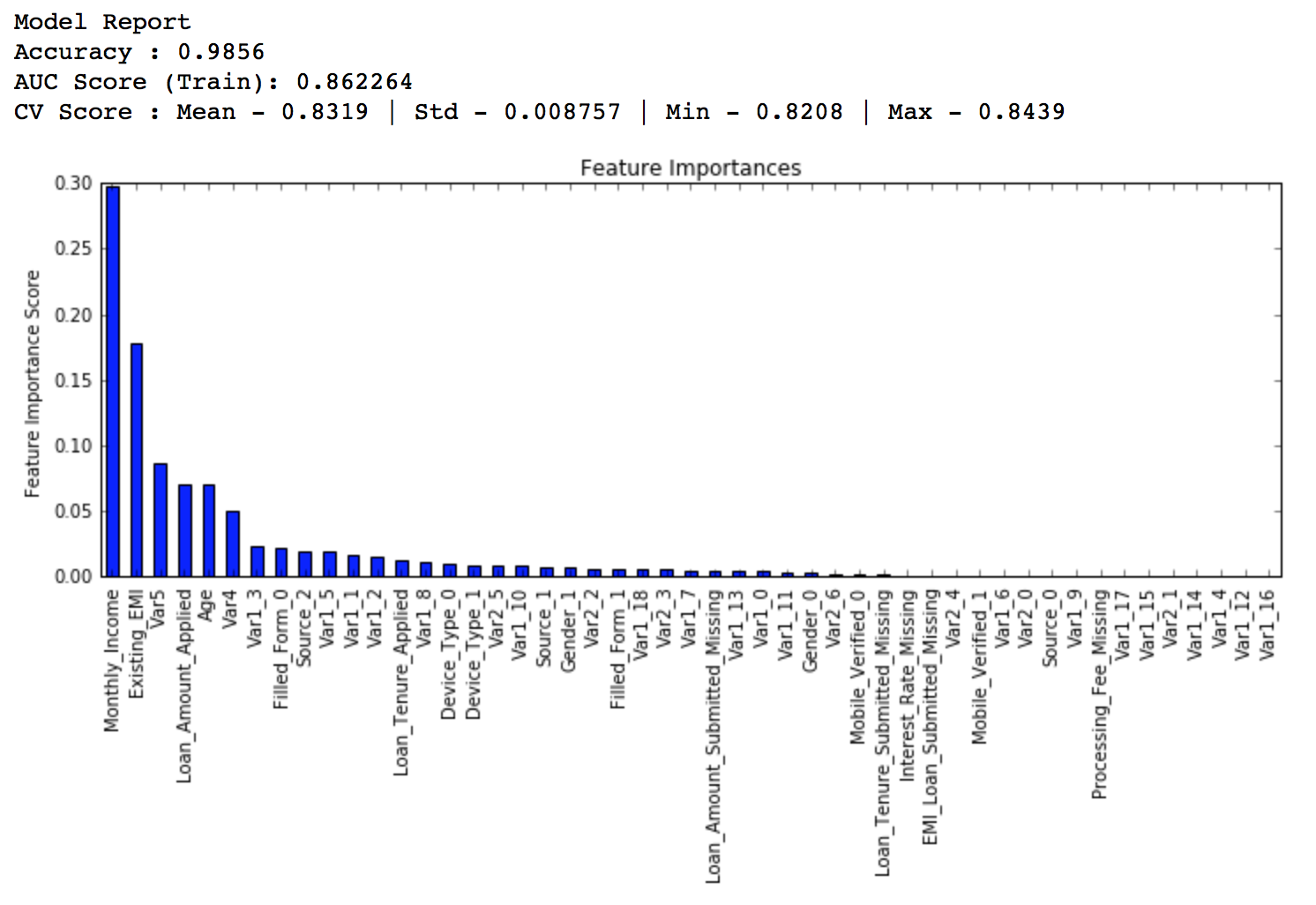### 5.1 参数调节的一般方法

1. 选择一个相对来说稍微高一点的learning rate。一般默认的值是0.1，不过针对不同的问题，0.05到0.2之间都可以
2. 决定当前learning rate下最优的决定树数量。它的值应该在40-70之间。记得选择一个你的电脑还能快速运行的值，因为之后这些树会用来做很多测试和调参。
3. 接着调节树参数来调整learning rate和树的数量。我们可以选择不同的参数来定义一个决定树，后面会有这方面的例子
4. 降低learning rate，同时会增加相应的决定树数量使得模型更加稳健

### 5.2固定 learning rate和需要估测的决定树数量

1. min_ samples_ split=500: 这个值应该在总样本数的0.5-1%之间，由于我们研究的是不均等分类问题，我们可以取这个区间里一个比较小的数，500。
2. min_ samples_ leaf=50: 可以凭感觉选一个合适的数，只要不会造成过度拟合。同样因为不均等分类的原因，这里我们选择一个比较小的值。
3. max_ depth=8: 根据观察数和自变量数，这个值应该在5-8之间。这里我们的数据有87000行，49列，所以我们先选深度为8。
4. max_ features=’sqrt’: 经验上一般都选择平方根。
5. subsample=0.8: 开始的时候一般就用0.8

#Choose all predictors except target & IDcols
predictors = [x for x in train.columns if x not in [target, IDcol]]
param_test1 = {'n_estimators':range(20,81,10)}
gsearch1 = GridSearchCV(estimator = GradientBoostingClassifier(learning_rate=0.1, min_samples_split=500,min_samples_leaf=50,max_depth=8,max_features='sqrt',subsample=0.8,random_state=10),
param_grid = param_test1, scoring='roc_auc',n_jobs=4,iid=False, cv=5)
gsearch1.fit(train[predictors],train[target])

gsearch1.grid_scores_, gsearch1.best_params_, gsearch1.best_score_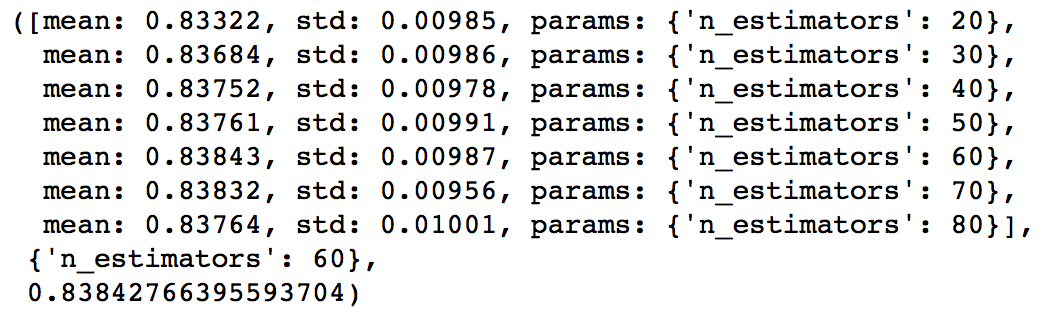1. 如果给出的输出是20，可能就要降低我们的learning rate到0.05，然后再搜索一遍。
2. 如果输出值太高，比如100，因为调节其他参数需要很长时间，这时候可以把learniing rate稍微调高一点。

### 5.3 调节树参数

1. 调节max_depth和 num_samples_split
2. 调节min_samples_leaf
3. 调节max_features

max_depth可以相隔两个数从5测到15，而min_samples_split可以按相隔200从200测到1000。这些完全凭经验和直觉，如果先测更大的范围再用迭代去缩小范围也是可行的。

param_test2 = {'max_depth':range(5,16,2), 'min_samples_split':range(200,1001,200)}
gsearch2 = GridSearchCV(estimator = GradientBoostingClassifier(learning_rate=0.1, n_estimators=60, max_features='sqrt', subsample=0.8, random_state=10),
param_grid = param_test2, scoring='roc_auc',n_jobs=4,iid=False, cv=5)
gsearch2.fit(train[predictors],train[target])
gsearch2.grid_scores_, gsearch2.best_params_, gsearch2.best_score_param_test3 = {'min_samples_split':range(1000,2100,200), 'min_samples_leaf':range(30,71,10)}
gsearch3 = GridSearchCV(estimator = GradientBoostingClassifier(learning_rate=0.1, n_estimators=60,max_depth=9,max_features='sqrt', subsample=0.8, random_state=10),
param_grid = param_test3, scoring='roc_auc',n_jobs=4,iid=False, cv=5)
gsearch3.fit(train[predictors],train[target])
gsearch3.grid_scores_, gsearch3.best_params_, gsearch3.best_score_modelfit(gsearch3.best_estimator_, train, predictors)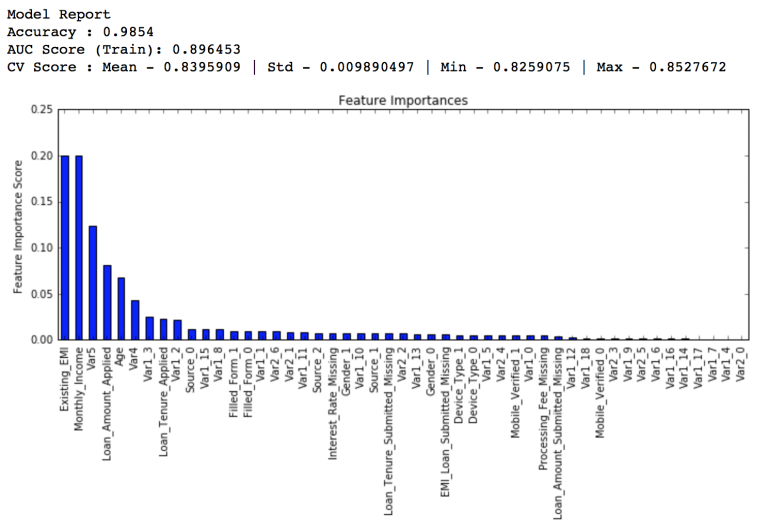param_test4 = {'max_features':range(7,20,2)}
gsearch4 = GridSearchCV(estimator = GradientBoostingClassifier(learning_rate=0.1, n_estimators=60,max_depth=9, min_samples_split=1200, min_samples_leaf=60, subsample=0.8, random_state=10),
param_grid = param_test4, scoring='roc_auc',n_jobs=4,iid=False, cv=5)
gsearch4.fit(train[predictors],train[target])
gsearch4.grid_scores_, gsearch4.best_params_, gsearch4.best_score_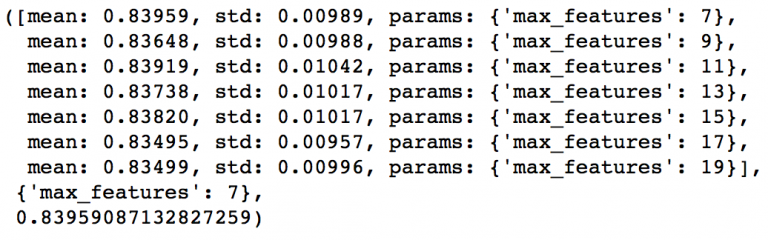• min_samples_split: 1200
• min_samples_leaf: 60
• max_depth: 9
• max_features: 7

### 5.4 调节子样本比例来降低learning rate

param_test5 = {'subsample':[0.6,0.7,0.75,0.8,0.85,0.9]}
gsearch5 = GridSearchCV(estimator = GradientBoostingClassifier(learning_rate=0.1, n_estimators=60,max_depth=9,min_samples_split=1200, min_samples_leaf=60, subsample=0.8, random_state=10,max_features=7),
param_grid = param_test5, scoring='roc_auc',n_jobs=4,iid=False, cv=5)
gsearch5.fit(train[predictors],train[target])
gsearch5.grid_scores_, gsearch5.best_params_, gsearch5.best_score_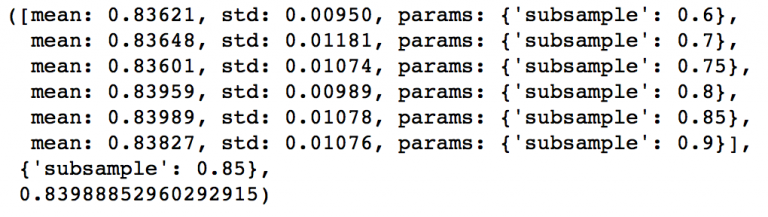predictors = [x for x in train.columns if x not in [target, IDcol]]
gbm_tuned_1 = GradientBoostingClassifier(learning_rate=0.05, n_estimators=120,max_depth=9, min_samples_split=1200,min_samples_leaf=60, subsample=0.85, random_state=10, max_features=7)
modelfit(gbm_tuned_1, train, predictors)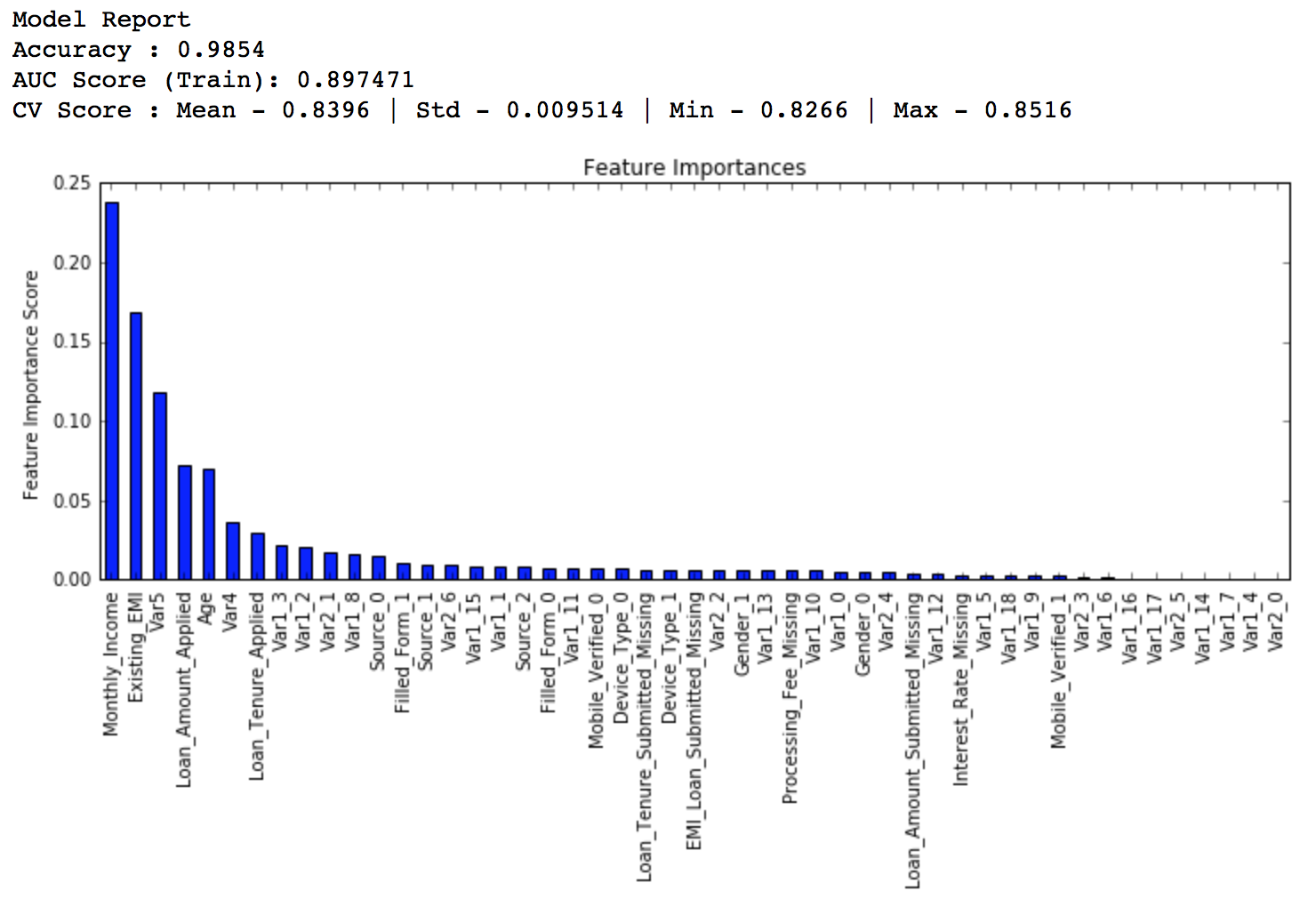predictors = [x for x in train.columns if x not in [target, IDcol]]
gbm_tuned_2 = GradientBoostingClassifier(learning_rate=0.01, n_estimators=600,max_depth=9, min_samples_split=1200,min_samples_leaf=60, subsample=0.85, random_state=10, max_features=7)
modelfit(gbm_tuned_2, train, predictors)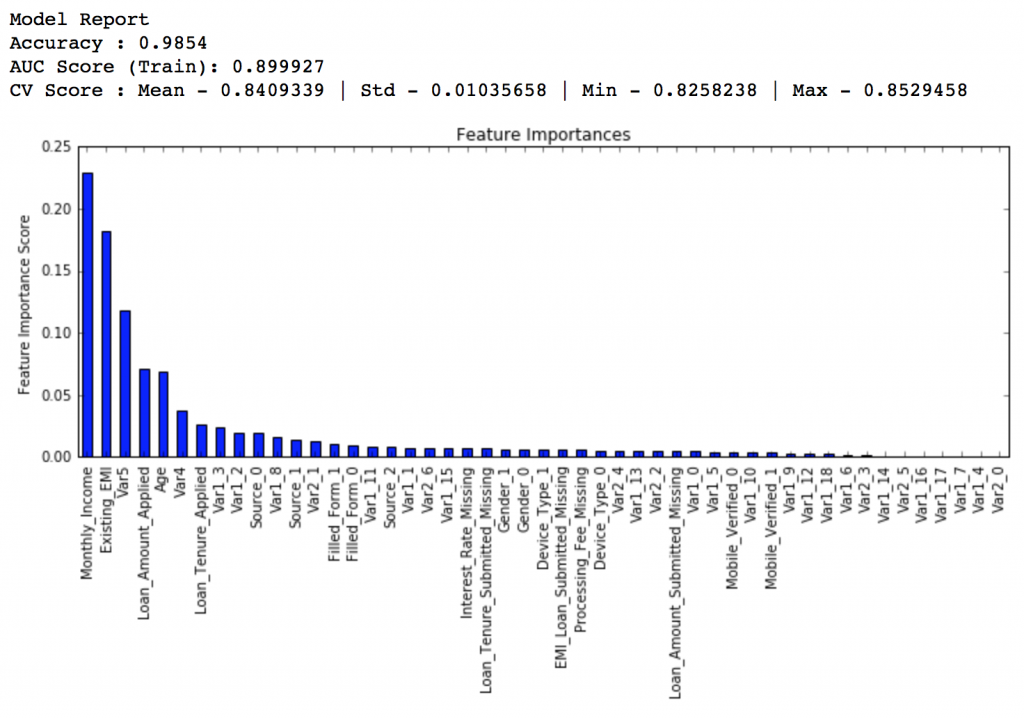predictors = [x for x in train.columns if x not in [target, IDcol]]
gbm_tuned_3 = GradientBoostingClassifier(learning_rate=0.005, n_estimators=1200,max_depth=9, min_samples_split=1200, min_samples_leaf=60, subsample=0.85, random_state=10, max_features=7,
warm_start=True)
modelfit(gbm_tuned_3, train, predictors, performCV=False)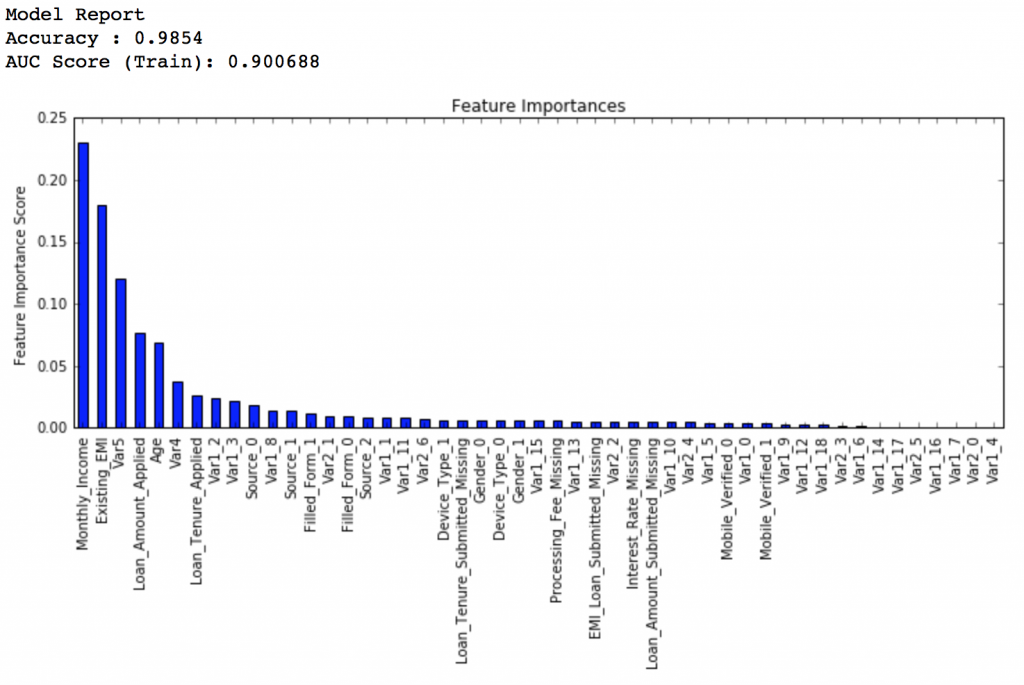predictors = [x for x in train.columns if x not in [target, IDcol]]
gbm_tuned_4 = GradientBoostingClassifier(learning_rate=0.005, n_estimators=1500,max_depth=9, min_samples_split=1200, min_samples_leaf=60, subsample=0.85, random_state=10, max_features=7,
warm_start=True)
modelfit(gbm_tuned_4, train, predictors, performCV=False)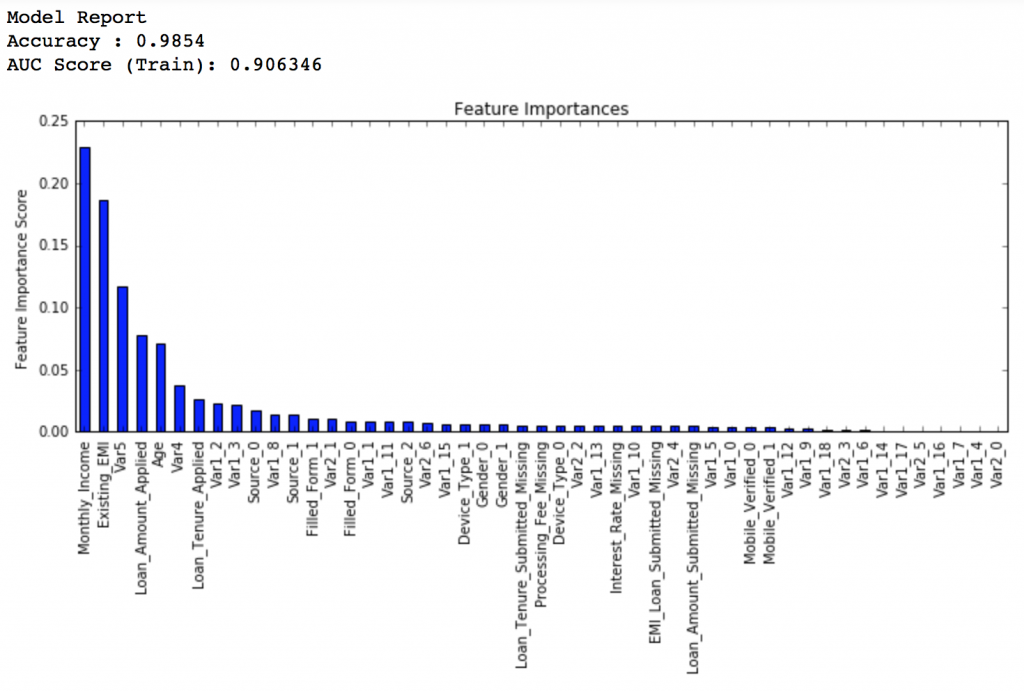## 6.总结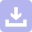下载
OptGBM：Optuna + LightGBM = OptGBM
02-15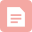博客

12-221448博客
scikit-learn 梯度提升(GBDT)调参小结
12-09937博客

03-246万+博客

12-041359博客

12-142143博客

05-167983博客
03-04138博客
10-249157博客

11-077752博客

08-19337博客
12-18165博客
Bagging和Boosting 概念及区别
06-30354博客
03-274753博客
08-033893博客

06-11350博客

10-24577博客

06-3099博客
boosting与bagging
01-24241

### “相关推荐”对你有帮助么？

•非常没帮助
•没帮助
•一般
•有帮助
•非常有帮助¥2 ¥4 ¥6 ¥10 ¥20余额支付 (余额：-- )扫码支付获取中扫码支付点击重新获取扫码支付1.余额是钱包充值的虚拟货币，按照1:1的比例进行支付金额的抵扣。
2.余额无法直接购买下载，可以购买VIP、C币套餐、付费专栏及课程。余额充值